$$\require{cancel}$$

# 9.E: Circuits (Exercises)

•• Contributed by Benjamin Crowell
• Professor (Physics) at Fullerton College

1. In a wire carrying a current of 1.0 pA, how long do you have to wait, on the average, for the next electron to pass a given point? Express your answer in units of microseconds. (solution in the pdf version of the book)a / Problem 2.

2. Referring back to our old friend the neuron from problem 1 on page 504, let's now consider what happens when the nerve is stimulated to transmit information. When the blob at the top (the cell body) is stimulated, it causes Na$$^+$$ ions to rush into the top of the tail (axon). This electrical pulse will then travel down the axon, like a flame burning down from the end of a fuse, with the Na$$^+$$ ions at each point first going out and then coming back in. If $$10^{10}$$ Na$$^+$$ ions cross the cell membrane in 0.5 ms, what amount of current is created?

(answer check available at lightandmatter.com)

3. If a typical light bulb draws about 900 mA from a 110-V household circuit, what is its resistance? (Don't worry about the fact that it's alternating current.) (answer check available at lightandmatter.com)

4. (a) Express the power dissipated by a resistor in terms of $$R$$ and $$\Delta V$$ only, eliminating $$I$$. (answer check available at lightandmatter.com)
(b) Electrical receptacles in your home are mostly 110 V, but circuits for electric stoves, air conditioners, and washers and driers are usually 220 V. The two types of circuits have differently shaped receptacles. Suppose you rewire the plug of a drier so that it can be plugged in to a 110 V receptacle. The resistor that forms the heating element of the drier would normally draw 200 W. How much power does it actually draw now? (answer check available at lightandmatter.com)

5. Lightning discharges a cloud during an electrical storm. Suppose that the current in the lightning bolt varies with time as $$I=bt$$, where $$b$$ is a constant. Find the cloud's charge as a function of time. (answer check available at lightandmatter.com)

6. A resistor has a voltage difference $$\Delta V$$ across it, causing a current $$I$$ to flow.
(a) Find an equation for the power it dissipates as heat in terms of the variables $$I$$ and $$R$$ only, eliminating $$\Delta V$$. (answer check available at lightandmatter.com)
(b) If an electrical line coming to your house is to carry a given amount of current, interpret your equation from part a to explain whether the wire's resistance should be small, or large.

7. In AM (amplitude-modulated) radio, an audio signal $$f(t)$$ is multiplied by a sine wave $$\sin \omega t$$ in the megahertz frequency range. For simplicity, let's imagine that the transmitting antenna is a whip, and that charge goes back and forth between the top and bottom. Suppose that, during a certain time interval, the audio signal varies linearly with time, giving a charge $$q=(a+bt)\sin \omega t$$ at the top of the whip and $$-q$$ at the bottom. Find the current as a function of time. (answer check available at lightandmatter.com)b / Problem 8.

8. Problem 8 has been deleted.

9. Use the result of problem 38 on page 550 to find an equation for the voltage at a point in space at a distance $$r$$ from a point charge $$Q$$. (Take your $$V=0$$ distance to be anywhere you like.) (answer check available at lightandmatter.com)

10. Today, even a big luxury car like a Cadillac can have an electrical system that is relatively low in power, since it doesn't need to do much more than run headlights, power windows, etc. In the near future, however, manufacturers plan to start making cars with electrical systems about five times more powerful. This will allow certain energy-wasting parts like the water pump to be run on electrical motors and turned off when they're not needed --- currently they're run directly on shafts from the motor, so they can't be shut off. It may even be possible to make an engine that can shut off at a stoplight and then turn back on again without cranking, since the valves can be electrically powered. Current cars' electrical systems have 12-volt batteries (with 14-volt chargers), but the new systems will have 36-volt batteries (with 42-volt chargers).
(a) Suppose the battery in a new car is used to run a device that requires the same amount of power as the corresponding device in the old car. Based on the sample figures above, how would the currents handled by the wires in one of the new cars compare with the currents in the old ones?
(b) The real purpose of the greater voltage is to handle devices that need more power. Can you guess why they decided to change to 36-volt batteries rather than increasing the power without increasing the voltage? (answer check available at lightandmatter.com)

11. We have referred to resistors dissipating heat, i.e., we have assumed that $$P=I\Delta V$$ is always greater than zero. Could $$I\Delta V$$ come out to be negative for a resistor? If so, could one make a refrigerator by hooking up a resistor in such a way that it absorbed heat instead of dissipating it?

12. What resistance values can be created by combining a 1 $$\text{k}\Omega$$ resistor and a 10 $$\text{k}\Omega$$ resistor? (solution in the pdf version of the book)

13. The figure shows a circuit containing five lightbulbs connected to a battery. Suppose you're going to connect one probe of a voltmeter to the circuit at the point marked with a dot. How many unique, nonzero voltage differences could you measure by connecting the other probe to other wires in the circuit?c / Problems 13 and 14.

14. The lightbulbs in the figure are all identical. If you were inserting an ammeter at various places in the circuit, how many unique currents could you measure? If you know that the current measurement will give the same number in more than one place, only count that as one unique current.

15. (a) You take an LP record out of its sleeve, and it acquires a static charge of 1 nC. You play it at the normal speed of $$33\frac{1}{3}$$ r.p.m., and the charge moving in a circle creates an electric current. What is the current, in amperes? (answer check available at lightandmatter.com)
(b) Although the planetary model of the atom can be made to work with any value for the radius of the electrons' orbits, more advanced models that we will study later in this course predict definite radii. If the electron is imagined as circling around the proton at a speed of $$2.2\times10^6$$ m/s, in an orbit with a radius of 0.05 nm, what electric current is created? (answer check available at lightandmatter.com)d / Problem 16.

16. The figure shows a simplified diagram of a device called a tandem accelerator, used for accelerating beams of ions up to speeds on the order of 1% of the speed of light. The nuclei of these ions collide with the nuclei of atoms in a target, producing nuclear reactions for experiments studying the structure of nuclei. The outer shell of the accelerator is a conductor at zero voltage (i.e., the same voltage as the Earth). The electrode at the center, known as the “terminal,” is at a high positive voltage, perhaps millions of volts. Negative ions with a charge of $$-1$$ unit (i.e., atoms with one extra electron) are produced offstage on the right, typically by chemical reactions with cesium, which is a chemical element that has a strong tendency to give away electrons. Relatively weak electric and magnetic forces are used to transport these $$-1$$ ions into the accelerator, where they are attracted to the terminal. Although the center of the terminal has a hole in it to let the ions pass through, there is a very thin carbon foil there that they must physically penetrate. Passing through the foil strips off some number of electrons, changing the atom into a positive ion, with a charge of $$+n$$ times the fundamental charge. Now that the atom is positive, it is repelled by the terminal, and accelerates some more on its way out of the accelerator.
(a) Find the velocity, $$v$$, of the emerging beam of positive ions, in terms of $$n$$, their mass $$m$$, the terminal voltage $$V$$, and fundamental constants. Neglect the small change in mass caused by the loss of electrons in the stripper foil.
(b) To fuse protons with protons, a minimum beam velocity of about 11% of the speed of light is required. What terminal voltage would be needed in this case? (answer check available at lightandmatter.com)
(c) In the setup described in part b, we need a target containing atoms whose nuclei are single protons, i.e., a target made of hydrogen. Since hydrogen is a gas, and we want a foil for our target, we have to use a hydrogen compound, such as a plastic. Discuss what effect this would have on the experiment. (answer check available at lightandmatter.com)

17. Wire is sold in a series of standard diameters, called “gauges.” The difference in diameter between one gauge and the next in the series is about 20%. How would the resistance of a given length of wire compare with the resistance of the same length of wire in the next gauge in the series? (answer check available at lightandmatter.com)e / Problem 18.

18. In the figure, the battery is 9 V.
(a) What are the voltage differences across each light bulb?(answer check available at lightandmatter.com)
(b) What current flows through each of the three components of the circuit? (answer check available at lightandmatter.com)
(c) If a new wire is added to connect points A and B, how will the appearances of the bulbs change? What will be the new voltages and currents?
(d) Suppose no wire is connected from A to B, but the two bulbs are switched. How will the results compare with the results from the original setup as drawn?f / Problem 19.

19. A student in a biology lab is given the following instructions: “Connect the cerebral eraser (C.E.) and the neural depolarizer (N.D.) in parallel with the power supply (P.S.). (Under no circumstances should you ever allow the cerebral eraser to come within 20 cm of your head.) Connect a voltmeter to measure the voltage across the cerebral eraser, and also insert an ammeter in the circuit so that you can make sure you don't put more than 100 mA through the neural depolarizer.” The diagrams show two lab groups' attempts to follow the instructions.
(a) Translate diagram 1 into a standard-style schematic. What is correct and incorrect about this group's setup?
(b) Do the same for diagram 2.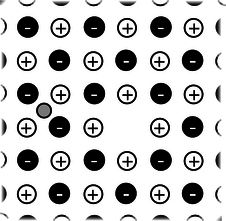g / Problem 20.

20. Referring back to problem 15 on page 506 about the sodium chloride crystal, suppose the lithium ion is going to jump from the gap it is occupying to one of the four closest neighboring gaps. Which one will it jump to, and if it starts from rest, how fast will it be going by the time it gets there? (It will keep on moving and accelerating after that, but that does not concern us.) [Hint: The approach is similar to the one used for the other problem, but you want to work with voltage and electrical energy rather than force.] (answer check available at lightandmatter.com)

21. A 1.0 $$\Omega$$ toaster and a 2.0 $$\Omega$$ lamp are connected in parallel with the 110-V supply of your house. (Ignore the fact that the voltage is AC rather than DC.)
(a) Draw a schematic of the circuit.
(b) For each of the three components in the circuit, find the current passing through it and the voltage drop across it. (answer check available at lightandmatter.com)
(c) Suppose they were instead hooked up in series. Draw a schematic and calculate the same things.(answer check available at lightandmatter.com)

22. The heating element of an electric stove is connected in series with a switch that opens and closes many times per second. When you turn the knob up for more power, the fraction of the time that the switch is closed increases. Suppose someone suggests a simpler alternative for controlling the power by putting the heating element in series with a variable resistor controlled by the knob. (With the knob turned all the way clockwise, the variable resistor's resistance is nearly zero, and when it's all the way counterclockwise, its resistance is essentially infinite.) (a) Draw schematics. (b) Why would the simpler design be undesirable?

23. You have a circuit consisting of two unknown resistors in series, and a second circuit consisting of two unknown resistors in parallel.
(a) What, if anything, would you learn about the resistors in the series circuit by finding that the currents through them were equal?
(b) What if you found out the voltage differences across the resistors in the series circuit were equal?
(c) What would you learn about the resistors in the parallel circuit from knowing that the currents were equal?
(d) What if the voltages in the parallel circuit were equal?

24. How many different resistance values can be created by combining three unequal resistors? (Don't count possibilities in which not all the resistors are used, i.e., ones in which there is zero current in one or more of them.)

25. Suppose six identical resistors, each with resistance $$R$$, are connected so that they form the edges of a tetrahedron (a pyramid with three sides in addition to the base, i.e., one less side than an Egyptian pyramid). What resistance value or values can be obtained by making connections onto any two points on this arrangement? (solution in the pdf version of the book)

26. A person in a rural area who has no electricity runs an extremely long extension cord to a friend's house down the road so she can run an electric light. The cord is so long that its resistance, $$x$$, is not negligible. Show that the lamp's brightness is greatest if its resistance, $$y$$, is equal to $$x$$. Explain physically why the lamp is dim for values of $$y$$ that are too small or too large.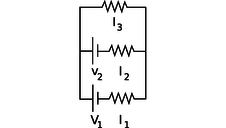h / Problem 27.

27. All three resistors have the same resistance, $$R$$. Find the three unknown currents in terms of $$V_1$$, $$V_2$$, and $$R$$. (answer check available at lightandmatter.com)i / Problem 28.

28. You are given a battery, a flashlight bulb, and a single piece of wire. Draw at least two configurations of these items that would result in lighting up the bulb, and at least two that would not light it. (Don't draw schematics.) If you're not sure what's going on, borrow the materials from your instructor and try it. Note that the bulb has two electrical contacts: one is the threaded metal jacket, and the other is the tip (at the bottom in the figure). [Problem by Arnold Arons.]j / Problem 29.

29. The figure shows a simplified diagram of an electron gun such as the one that creates the electron beam in a TV tube. Electrons that spontaneously emerge from the negative electrode (cathode) are then accelerated to the positive electrode, which has a hole in it. (Once they emerge through the hole, they will slow down. However, if the two electrodes are fairly close together, this slowing down is a small effect, because the attractive and repulsive forces experienced by the electron tend to cancel.)
(a) If the voltage difference between the electrodes is $$\Delta V$$, what is the velocity of an electron as it emerges at B? Assume that its initial velocity, at A, is negligible, and that the velocity is nonrelativistic. (If you haven't read ch. 7 yet, don't worry about the remark about relativity.) (answer check available at lightandmatter.com)
(b) Evaluate your expression numerically for the case where $$\Delta V$$=10 kV, and compare to the speed of light. If you've read ch. 7 already, comment on whether the assumption of nonrelativistic motion was justified. (solution in the pdf version of the book)(answer check available at lightandmatter.com)

30. (a) Many battery-operated devices take more than one battery. If you look closely in the battery compartment, you will see that the batteries are wired in series. Consider a flashlight circuit. What does the loop rule tell you about the effect of putting several batteries in series in this way?
(b) The cells of an electric eel's nervous system are not that different from ours --- each cell can develop a voltage difference across it of somewhere on the order of one volt. How, then, do you think an electric eel can create voltages of thousands of volts between different parts of its body?k / Problem 31.

31. The figure shows two possible ways of wiring a flashlight with a switch. Both will serve to turn the bulb on and off, although the switch functions in the opposite sense. Why is method (1) preferable?l / A printed circuit board, like the kind referred to in problem 32.

32. You have to do different things with a circuit to measure current than to measure a voltage difference. Which would be more practical for a printed circuit board, in which the wires are actually strips of metal embedded inside the board? (solution in the pdf version of the book)m / Problem 33.

33. The bulbs are all identical. Which one doesn't light up?n / Problem 34.

34. Each bulb has a resistance of one ohm. How much power is drawn from the one-volt battery? (answer check available at lightandmatter.com)o / Problem 35.

35. The bulbs all have unequal resistances. Given the three currents shown in the figure, find the currents through bulbs A, B, C, and D.

36. A silk thread is uniformly charged by rubbing it with llama fur. The thread is then dangled vertically above a metal plate and released. As each part of the thread makes contact with the conducting plate, its charge is deposited onto the plate. Since the thread is accelerating due to gravity, the rate of charge deposition increases with time, and by time $$t$$ the cumulative amount of charge is $$q=ct^2$$, where $$c$$ is a constant. (a) Find the current flowing onto the plate.(answer check available at lightandmatter.com)
(b) Suppose that the charge is immediately carried away through a resistance $$R$$. Find the power dissipated as heat.(answer check available at lightandmatter.com)

37. In example 9 on p. 524, suppose that the larger sphere has radius $$a$$, the smaller one $$b$$. (a) Use the result of problem 9 show that the ratio of the charges on the two spheres is $$q_a/q_b=a/b$$. (b) Show that the density of charge (charge per unit area) is the other way around: the charge density on the smaller sphere is greater than that on the larger sphere in the ratio $$a/b$$.

38. (a) Recall that the gravitational energy of two gravitationally interacting spheres is given by $$PE=-Gm_1m_2/r$$, where $$r$$ is the center-to-center distance. Sketch a graph of $$PE$$ as a function of $$r$$, making sure that your graph behaves properly at small values of $$r$$, where you're dividing by a small number, and at large ones, where you're dividing by a large one. Check that your graph behaves properly when a rock is dropped from a larger $$r$$ to a smaller one; the rock should lose potential energy as it gains kinetic energy.
(b) Electrical forces are closely analogous to gravitational ones, since both depend on $$1/r^2$$. Since the forces are analogous, the potential energies should also behave analogously. Using this analogy, write down the expression for the electrical potential energy of two interacting charged particles. The main uncertainty here is the sign out in front. Like masses attract, but like charges repel. To figure out whether you have the right sign in your equation, sketch graphs in the case where both charges are positive, and also in the case where one is positive and one negative; make sure that in both cases, when the charges are released near one another, their motion causes them to lose PE while gaining KE.(answer check available at lightandmatter.com)p / Problem 39.

39. Find the current drawn from the battery. (answer check available at lightandmatter.com)

# Exercises

## Exercise A: Voltage and Current

1. How many different currents could you measure in this circuit? Make a prediction, and then try it.What do you notice? How does this make sense in terms of the roller coaster metaphor introduced in discussion question 9.1.3A on page 519?

What is being used up in the resistor?

2. By connecting probes to these points, how many ways could you measure a voltage? How many of them would be different numbers? Make a prediction, and then do it.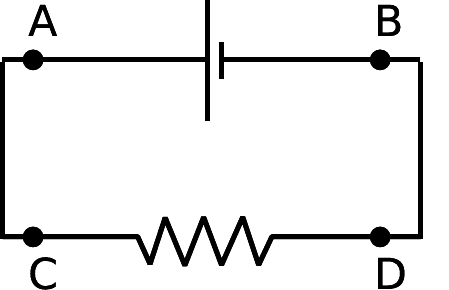What do you notice? Interpret this using the roller coaster metaphor, and color in parts of the circuit that represent constant voltages.

3. The resistors are unequal. How many different voltages and currents can you measure? Make a prediction, and then try it.What do you notice? Interpret this using the roller coaster metaphor, and color in parts of the circuit that represent constant voltages.

## Exercise B: The Loop and Junction Rules

Apparatus:

• DC power supply
• multimeter
• resistors

1. The junction rule

Construct a circuit like this one, using the power supply as your voltage source. To make things more interesting, don't use equal resistors. Use nice big resistors (say 100 $$k\Omega$$ to 1 $$M\Omega$$) --- this will ensure that you don't burn up the resistors, and that the multimeter's small internal resistance when used as an ammeter is negligible in comparison.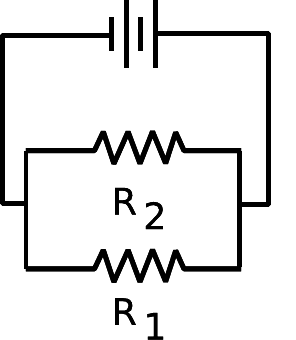Insert your multimeter in the circuit to measure all three currents that you need in order to test the junction rule.

2. The loop rule

Now come up with a circuit to test the loop rule. Since the loop rule is always supposed to be true, it's hard to go wrong here! Make sure you have at least three resistors in a loop, and make sure you hook in the power supply in a way that creates non-zero voltage differences across all the resistors. Measure the voltage differences you need to measure to test the loop rule. Here it is best to use fairly small resistances, so that the multimeter's large internal resistance when used in parallel as a voltmeter will not significantly reduce the resistance of the circuit. Do not use resistances of less than about 100 $$\Omega$$, however, or you may blow a fuse or burn up a resistor.

## Exercise C: Reasoning About Circuits

The questions in this exercise can all be solved using some combination of the following approaches:

a) There is constant voltage throughout any conductor.

b) Ohm's law can be applied to any part of a circuit.

c) Apply the loop rule.

d) Apply the junction rule.

In each case, discuss the question, decide what you think is the right answer, and then try the experiment.

1. A wire is added in parallel with one bulb.Which reasoning is correct?

• Each bulb still has 1.2 V across it, so both bulbs are still lit up.
• All parts of a wire are at the same voltage, and there is now a wire connection from one side of the right-hand bulb to the other. The right-hand bulb has no voltage difference across it, so it goes out.

2. The series circuit is changed as shown.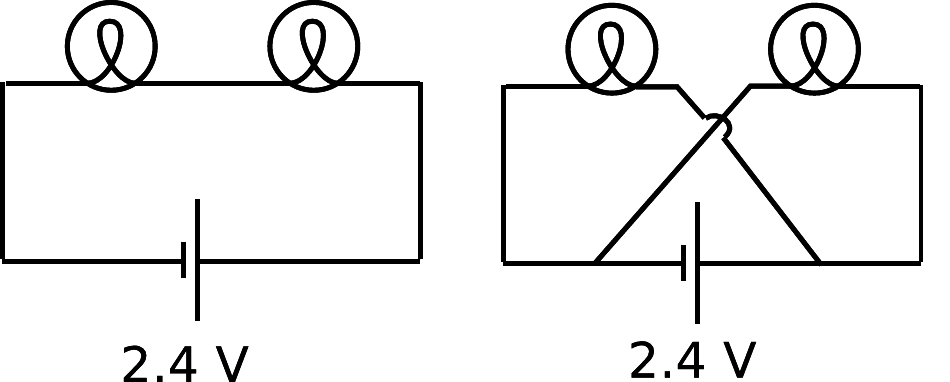Which reasoning is correct?

• Each bulb now has its sides connected to the two terminals of the battery, so each now has 2.4 V across it instead of 1.2 V. They get brighter.
• Just as in the original circuit, the current goes through one bulb, then the other. It's just that now the current goes in a figure-8 pattern. The bulbs glow the same as before.

3. A wire is added as shown to the original circuit.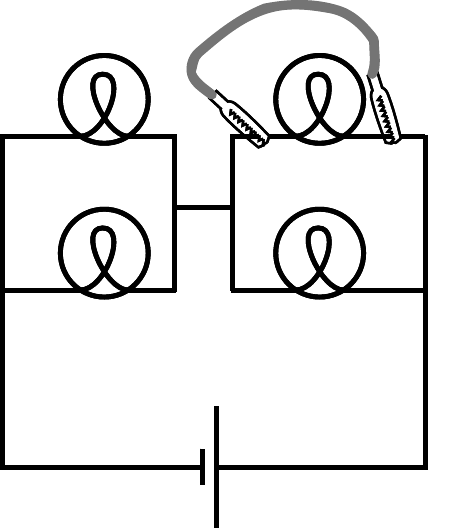What is wrong with the following reasoning?

The top right bulb will go out, because its two sides are now connected with wire, so there will be no voltage difference across it. The other three bulbs will not be affected.

4. A wire is added as shown to the original circuit.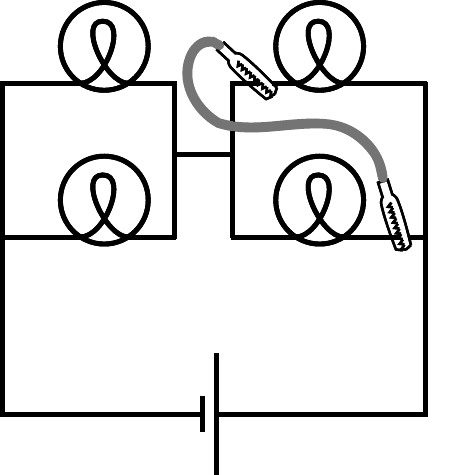What is wrong with the following reasoning?

The current flows out of the right side of the battery. When it hits the first junction, some of it will go left and some will keep going up The part that goes up lights the top right bulb. The part that turns left then follows the path of least resistance, going through the new wire instead of the bottom bulb. The top bulb stays lit, the bottom one goes out, and others stay the same.

5. What happens when one bulb is unscrewed, leaving an air gap?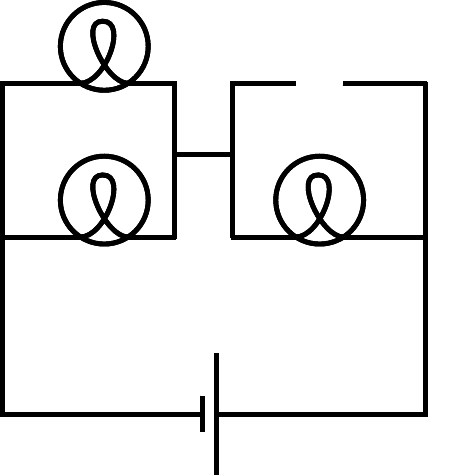(c) 1998-2013 Benjamin Crowell, licensed under the Creative Commons Attribution-ShareAlike license. Photo credits are given at the end of the Adobe Acrobat version.

## Contributors

Benjamin Crowell (Fullerton College). Conceptual Physics is copyrighted with a CC-BY-SA license.